# Probability outcomes for coins, dice, and spinners#### All in One Place

Everything you need for JC, LC, and college level maths and science classes.#### Learn with Ease

We’ve mastered the national curriculum so that you can revise with confidence.#### Instant Help

24/7 access to the best tips, walkthroughs, and practice exercises available.

0/2
##### Intros
###### Lessons
1. Introduction to Probability Outcomes for Coins, Dice, and Spinners:
2. What is probability?
3. What are outcomes in probability?
1. Outcomes for flipping a coin
2. Outcomes for rolling a 6-sided die
3. Outcomes for a spinner with different regions
0/7
##### Examples
###### Lessons
1. Outcomes are the result of flipping coins, rolling dice, or turning a spinner's arrow
List all the possible outcomes. How many outcomes are there in total?
1. A coin with a happy face or sad face on its sides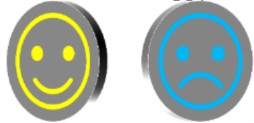2. An 8-sided die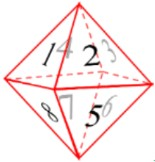3. Two friends having a race against each other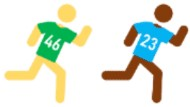2. Outcomes that are equally as likely to occur
Fill in the table for the probability outcomes
1. Rolling a 6-sided die: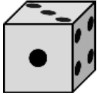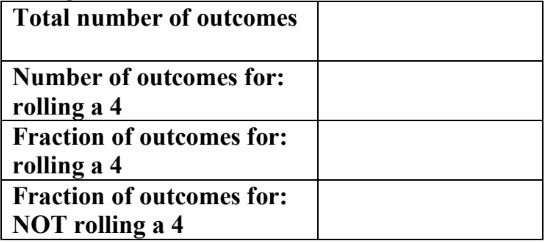2. Turning a spinner with letters: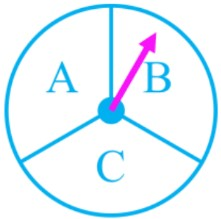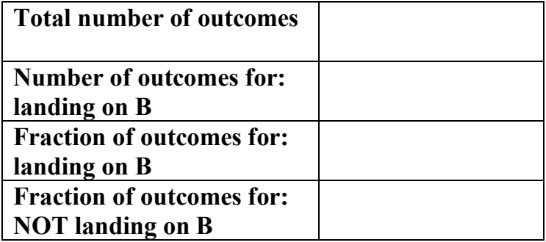3. Turning a spinner with numbers: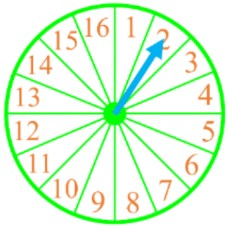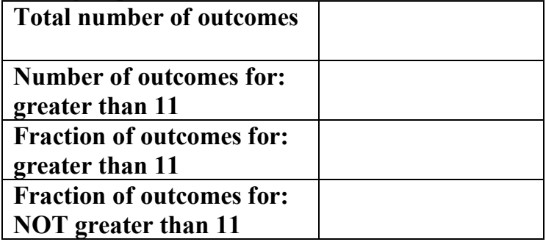4. Rolling a 6-sided die: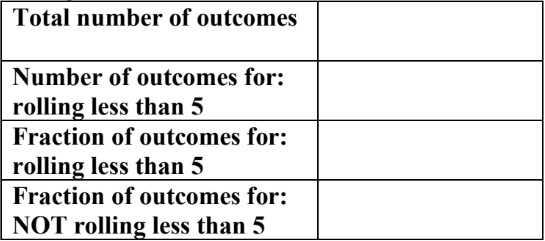###### Free to Join!
StudyPug is a learning help platform covering math and science from grade 4 all the way to second year university. Our video tutorials, unlimited practice problems, and step-by-step explanations provide you or your child with all the help you need to master concepts. On top of that, it's fun - with achievements, customizable avatars, and awards to keep you motivated.
• #### Easily See Your ProgressWe track the progress you've made on a topic so you know what you've done. From the course view you can easily see what topics have what and the progress you've made on them. Fill the rings to completely master that section or mouse over the icon to see more details.
• #### Make Use of Our Learning Aids###### Practice Accuracy

Get quick access to the topic you're currently learning.

See how well your practice sessions are going over time.

Stay on track with our daily recommendations.

• #### Earn Achievements as You LearnMake the most of your time as you use StudyPug to help you achieve your goals. Earn fun little badges the more you watch, practice, and use our service.
• #### Create and Customize Your AvatarPlay with our fun little avatar builder to create and customize your own avatar on StudyPug. Choose your face, eye colour, hair colour and style, and background. Unlock more options the more you use StudyPug.
###### Topic Notes

In this lesson, we will learn:

• What is probability in math?
• How to write probability as a fraction
• How to list all the outcomes for probability events (using coins, dice, and spinners)

Notes:

• Probability is math for events that only sometimes happen. The chances of something happening can be likely or unlikely to happen.
• Calculating probability is like predicting the future. We are trying to get a measure of the chances that something will happen.

• Arithmetic math (all basic operations; adding, subtracting, multiplying, dividing numbers) is certain. In comparison, probability math is uncertain--but still predictable.

• Probability can be given as a fraction, following the formula format:

• Probability = $\frac{number\,of\, outcomes\, wanted} {total\,number\,possible\,outcomes}$

• Outcomes are all the possible endings that could happen for a situation.
• Some simple probability situations that are often used in math problems include: tossing a coin, rolling a six-sided die, and spinning the arrow on a spinner.

• Coins: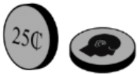• If you toss a coin, it will land on a flat side—either on heads or tails
• So, there are 2 possible outcomes to a coin toss: heads or tails
• The probability of landing on heads is $P$(heads) = $\frac{1}{2}$
• The probability of landing on heads is $P$(tails) = $\frac{1}{2}$

• Dice: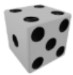(a six-sided die, if singular)
• When rolling a die, it will land with one of its six flat faces facing up (on top). There are six sides labelled from 1 to 6.
• So, there are 6 possible outcomes to a die roll: 1, 2, 3, 4, 5, or 6
• The probability of landing on any one side (1-6) is one out of six chances
• $P$ (1) =$P$ (2) = $P$ (3) = $P$ (4) = $P$ (5) = $P$ (6) = $\frac{1}{6}$

• Spinners: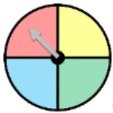(can have any number of equal parts; labels with numbers, letters, etc.)
• When using a spinner, the arrow will land on one of the marked regions. In this case, there are 4 different coloured regions.
• So, there are 4 possible outcomes for this spinner: red, yellow, green, or blue
• The probability of landing on any one of the colors is one out of four chances
• $P$ (Red) = $P$ (Yellow) = $P$ (Green) = $P$ (Blue) = $\frac{1}{4}$